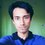# A Quick Probability Problem

Here I am with a short post. This is a short problem on probability. Post your solution in the comments section.

Calvin lies $$\frac{2}{3}$$ of the time and Peter lies $$\frac{1}{3}$$ of the time. I asked Calvin if he'd completed his homework. He said no. Then Peter said, "Calvin is telling the truth."

What is the probability that Calvin completed his homework? What is the probability that he didn't?

Oh yes, and "All characters appearing in this problem are fictitious. Any resemblance to real persons, living or dead, is purely coincidental." :)Note by Mursalin Habib
7 years, 6 months ago

This discussion board is a place to discuss our Daily Challenges and the math and science related to those challenges. Explanations are more than just a solution — they should explain the steps and thinking strategies that you used to obtain the solution. Comments should further the discussion of math and science.

When posting on Brilliant:

• Use the emojis to react to an explanation, whether you're congratulating a job well done , or just really confused .
• Ask specific questions about the challenge or the steps in somebody's explanation. Well-posed questions can add a lot to the discussion, but posting "I don't understand!" doesn't help anyone.
• Try to contribute something new to the discussion, whether it is an extension, generalization or other idea related to the challenge.

MarkdownAppears as
*italics* or _italics_ italics
**bold** or __bold__ bold
- bulleted- list
• bulleted
• list
1. numbered2. list
1. numbered
2. list
Note: you must add a full line of space before and after lists for them to show up correctly
paragraph 1paragraph 2

paragraph 1

paragraph 2

[example link](https://brilliant.org)example link
> This is a quote
This is a quote
    # I indented these lines
# 4 spaces, and now they show
# up as a code block.

print "hello world"
# I indented these lines
# 4 spaces, and now they show
# up as a code block.

print "hello world"
MathAppears as
Remember to wrap math in $$ ... $$ or $ ... $ to ensure proper formatting.
2 \times 3 $2 \times 3$
2^{34} $2^{34}$
a_{i-1} $a_{i-1}$
\frac{2}{3} $\frac{2}{3}$
\sqrt{2} $\sqrt{2}$
\sum_{i=1}^3 $\sum_{i=1}^3$
\sin \theta $\sin \theta$
\boxed{123} $\boxed{123}$

Sort by:

By Peter's statement, the truth of Calvin's statement is the same as the truth of Peter's statement (ie they're both lying or both telling the truth). The probability that they are both lying is $\frac{2}{3} \cdot \frac{1}{3} = \frac{2}{9}$. The probability that they are both telling the truth is $\frac{1}{3} \cdot \frac{2}{3} = \frac{2}{9}$.

We know that one of these cases must be true, and thus the probability that Calvin didn't complete his homework is,

$\frac{^2/_9}{^2/_9 + {}^2/_9} = \boxed{\frac{1}{2}}$

Is this right, and would this also work if the probabilities were unequal?

- 7 years, 6 months ago

$\frac{2/9}{2/9}=\frac{1}{2}$

What?!?

- 7 years, 6 months ago

I wasn't thinking. It should make more sense now...

It was supposed to be the probability of the desired event, divided by the sum of the probabilities of all allowed possibilities.

- 7 years, 6 months ago

I see what you did there. Can you find what's wrong with this argument?

Both Calvin & Peter said that Calvin didn't complete his homework. So, for the homework to be incomplete, both of their statements have to be true. But the probability that both of their statements are true is $\frac{2}{9}$. So, our desired probability is $\frac{2}{9}$.

- 7 years, 6 months ago

That argument is wrong, because it misses information that can be derived from Calvin and Peter's statements, which would change the probability (from the reader's perspective).

Namely, it can be seen that one person lying while the other is telling the truth would be a logical fallacy, and so they are either both lying or both telling the truth. Thus, you must compare the probability of the desired event to the probabilities of all possible events, discarding the impossible ones.

- 7 years, 6 months ago

Okay, now what's wrong with this argument?

If Calvin's statement is true, so is Peter's. The probability that Calvin's statement is true is $\frac{1}{3}$. So, the probability that he didn't complete his homework is simply $\frac{1}{3}$.

- 7 years, 6 months ago

Both have said the same answer "No", so either both are true or both are false. So, the event which has already occurred is that they have said the same thing which has the probability $\frac{4}{9} (\frac{1}{3} * \frac{2}{3} + \frac{2}{3} * \frac{1}{3})$. What we are required is that both spoke the truth which has probability $\frac{2}{3}$. So according to Bayes' theorem the answer is $\frac{\frac{2}{9}}{ \frac{1}{9} + \frac{2}{9}} = \boxed{\frac{1}{2}}$

- 7 years, 6 months ago

Typo: It's 2/9 instead of 1/9 in the denomination.

- 7 years, 6 months ago

denominator*

- 7 years, 6 months ago